# Asymmetrical conductor model with a hole (TEAM 7)

HOME / Applications / Asymmetrical conductor model with a hole (TEAM 7)
WEBINAR
Tips for Permanent Magnet Linear Generators Design
Thursday, October 21, 2021
Time
SESSION 1
SESSION 2
CEST (GMT +2)
03:00 PM
08:00 PM
EDT (GMT -4)
09:00 AM
02:00 PM
Used Tools: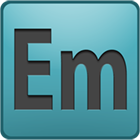## Description of the problem

The TEAM Benchmarks originate from the Argonne National Laboratory (ANL) in 1985 where a series of workshops started in 1986. In short, the goal of the workshops and ensuing benchmarks was to "show the effectiveness of numerical techniques and associated computer codes in solving electromagnetic field problems, and to gain confidence in their predictions. The workshops should also provide cooperation between workers, leading to an interchange of ideas".

The model shown below (Figure 1)consists of a thick aluminum plate with an eccentric hole and an exciting coil. Since the structure is asymmetrical, it is modeled in its entirety.

This problem is known as the TEAM Workshop problem #7.  Measured data and problem description are included in .  More measured results are presented in .  In the results section, a comparison with the measured results will be given.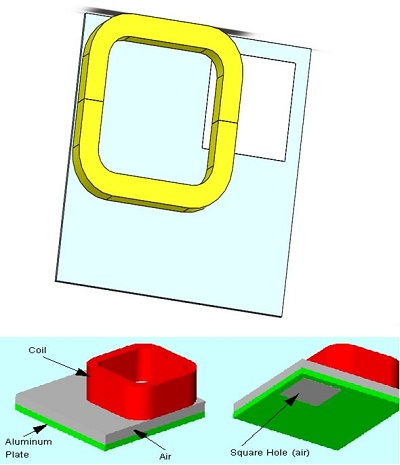Figure 1 - 3D model

## Skin Depth Calculation

The first step for AC Magnetic problems is always to calculate the skin depth (d) or the depth of penetration of the field for the conducting regions. For the current problem, the skin depth must be calculated in the Aluminum plate at a frequency f = 50 Hz,  , S/m Using the following equation.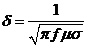We obtain d = 11.98 mm. The height of the Aluminum plate H = 19mm. Thus H/d = 19/11.98 = 1.58. Hence the current problem indeed requires the AC Magnetic analysis. Furthermore, as mentioned earlier, for the AC magnetic analysis, the mesh must have at least two elements per skin depth in the conducting regions where an eddy current is expected to be induced. Given a ratio of   H/d = 1.58, 3 to 4 mesh elements along the height of the Aluminum plate are sufficient.

Since the coil is stranded, it does not support eddy currents. Thus, the calculation of the skin depth in the coil is not needed.

## Study

The AC Magnetic module of EMS is used to compute and visualize magnetic fields. These fields are typically caused by surges in currents or voltages. This type of analysis can be linear or non-linear. It also addresses eddy currents, power losses and magnetic forces. After creating a AC Magnetic study and thermal analysis coupling in EMS, four important steps shall always be followed: 1 - apply the proper material for all solid bodies, 2- apply the necessary boundary conditions, or the so called Loads/Restraints in EMS, 3 - mesh the entire model and 4- run the solver.

## Materials

In the AC Magnetic  analysis of EMS,  the whole properties of material are needed (Table 1).

Table1 - Table of materials

 Components / Bodies Material Relative permeability Conductivity (S/m) Half Coil 1 / Half Coil 2 Copper 0.999991 5.7e+007 Outer Air / Airb Air 1 0 Plate Aluminum 1 3.526e+007 Hole Hole 1 1

## ElectroMagnetic Input

It is important to realize that the exciting coil in this example makes a closed loop, i.e. multiply-connected. Since the problem is asymmetric, the entire coil has to be included in the model.  The current density must flow orthogonal to the entry port.  To make such port  accessible for picking, the coil was divided to 2 parts Coil-1 and Coil2-1.   In addition, the entry and exit ports are the same.  Thus, only the entry port must be specified.
Table 2 -  coil information

 Name Number of turns Magnitude Phase Wound Coil 100 19.39 A 0

## Meshing

Meshing is a very crucial step in the design analysis. EMS estimates a global element size for the model taking into consideration its volume, surface area, and other geometric details. The size of the generated mesh (number of nodes and elements) depends on the geometry and dimensions of the model, element size, mesh tolerance, and mesh control. In the early stages of design analysis where approximate results may suffice, you can specify a larger element size for a faster solution. For a more accurate solution, a smaller element size may be required.

Mesh quality can be adjusted using Mesh Control (Table 3), which can be applied on solid bodies and faces. Below (Figure 4) is the meshed model after using Mesh Controls.

Table 3 -  Mesh control

 Name Mesh size Components /Bodies Mesh control 1 5 mm Plate Mesh control 1 10 mm Hole / Half coil 1/Half coil 2/ Airb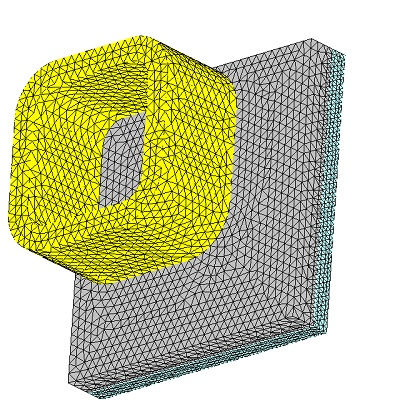Figure 2 - Meshed model

## Results

After running the simulation of this example we can obtain many results. AC Magnetic Module generates the results of : Magnetic Flux Density (Figure 3,4), Magnetic Field Intensity, Applied Current Density (Figure 5), Eddy Current Density (Figure 6 ), Force density, Losses Density (Figure 7) and a results table which contains the computed parameters of the model (Inductance, Current, Induced voltage, Losses etc ..) , the electromagnetic forces .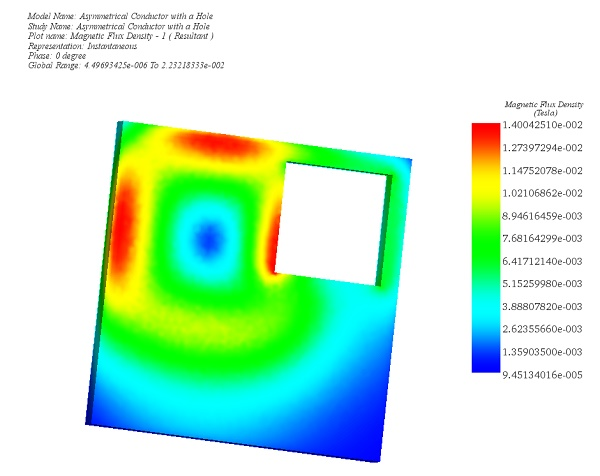Figure 3 - Magnetic Flux Density in the plate, fringe plot (Phase 0)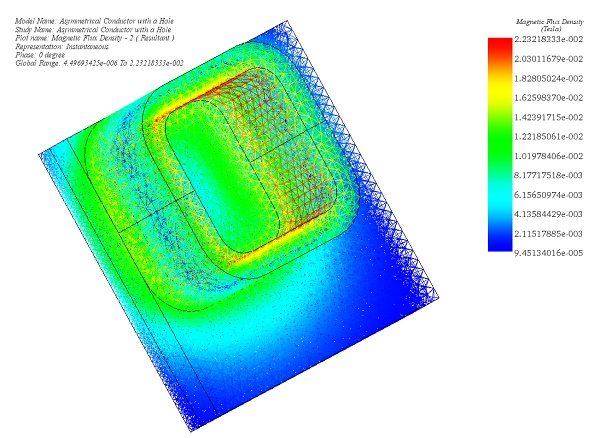Figure 4 - Magnetic Flux Density, mesh plot (Phase 0)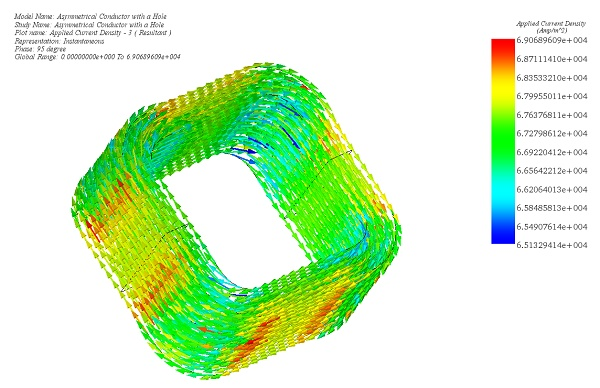Figure 5 - Applied Current Density, vector plot (Phase 95 deg)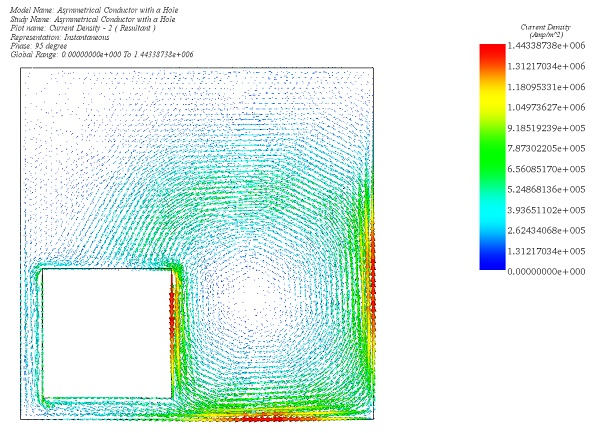Figure 6 - Eddy Current Density in the plate , vector plot (phase 95 deg)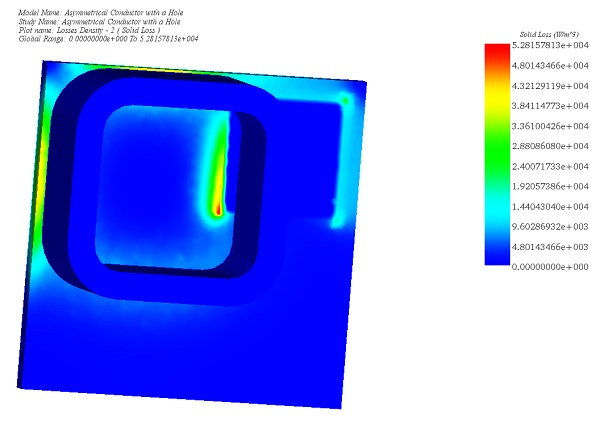Figure 7 - Solid Loss due to the Joule Effect

Among the benchmark results required by TEAM 7 is the plot of magnetic flux density along the Z-axis (Bz) through the line A1B1 described in the sketch below: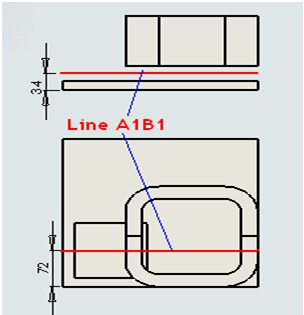The measured data is reported in  and .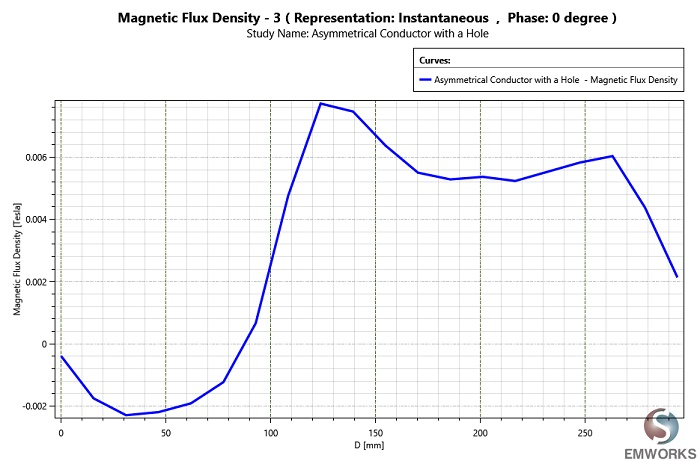The above results compare well to the measured data reported in  and .

## Conclusion

The above results compare well to the measured data reported in  and .

## References

 K. Fujiware and T. Nakata,  "Results for benchmark Problem 7 (asymmetric conductor with a hole),"  in Compel, vol. 9, no. 3,  pp. 137-154, 1990.
. Oszkar Biro and Kurt Preis, "An edge finite element eddy current formulation using a reduced magnetic and a current vector potential," IEEE Transactions on Magnetics, vol. 36, no. 5, pp. 3128-3130, September 2000.